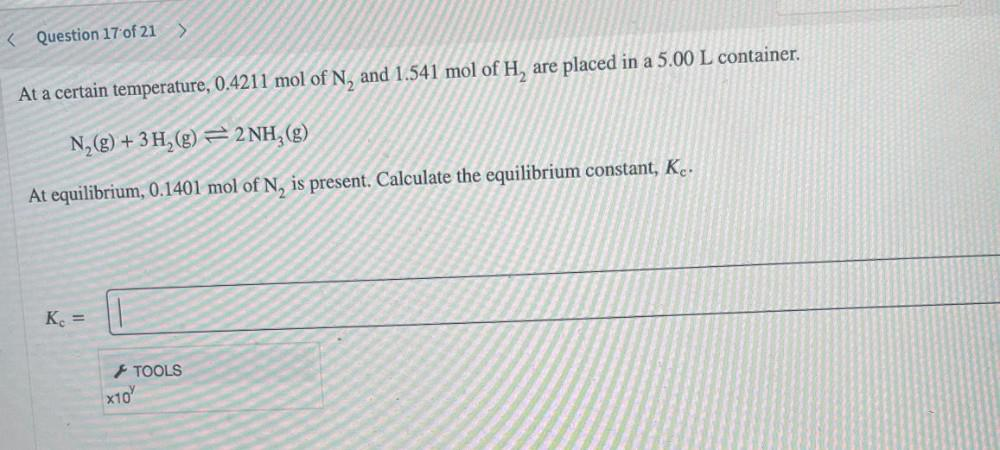Question:

# At a certain temperature, 0.4211 mol of N, and 1.541 mol of H, are placed in a 5.00 L container. N₂(g) + 3H₂ ⥨ 2NH3(g) At equilibrium, 0.1401 mol of N, is present. Calculate the equilibrium constant,At a certain temperature, 0.4211 mol of N, and 1.541 mol of H, are placed in a 5.00 L container. N₂(g) + 3H₂ ⥨ 2NH3(g) At equilibrium, 0.1401 mol of N, is present. Calculate the equilibrium constant, Kc.